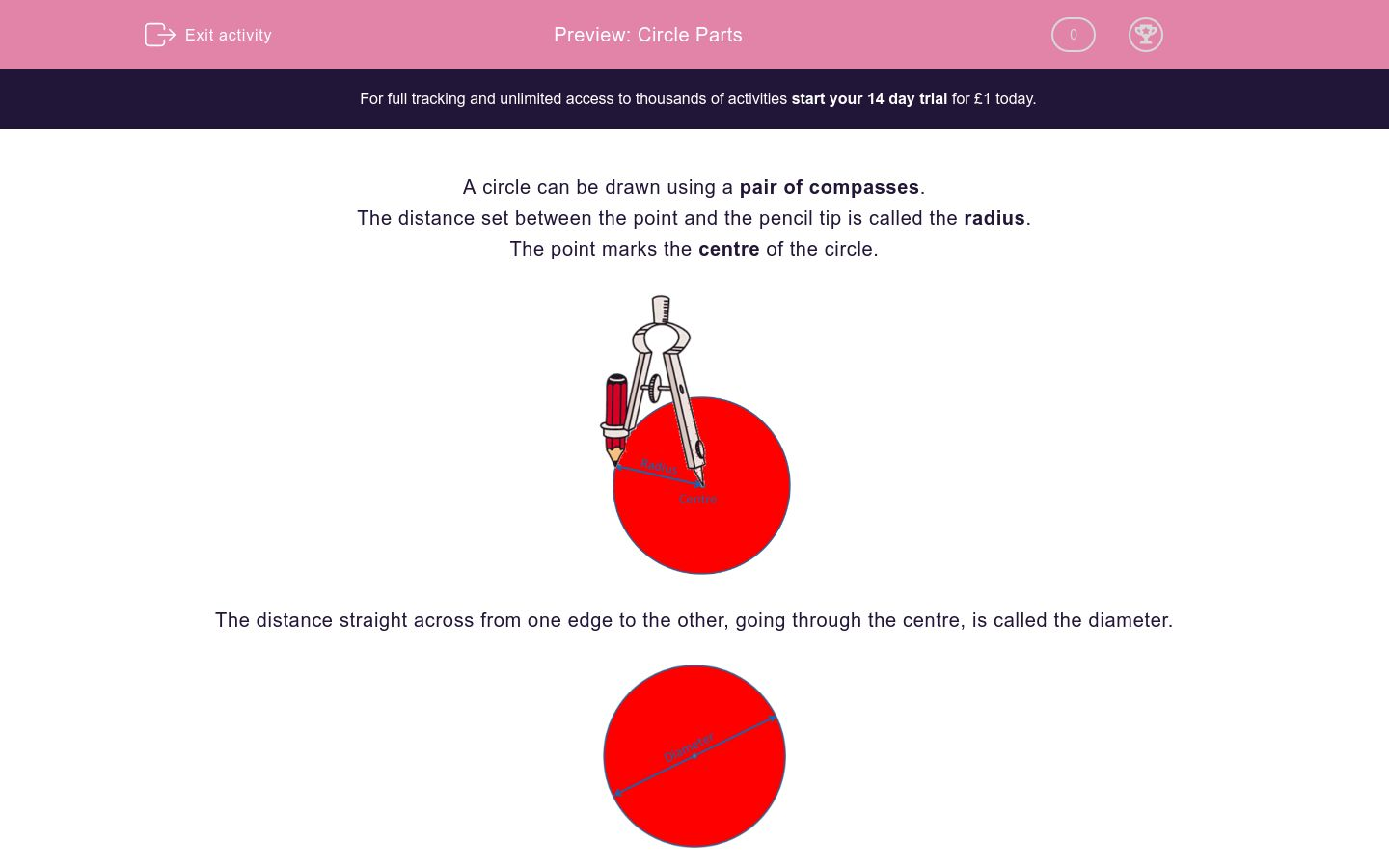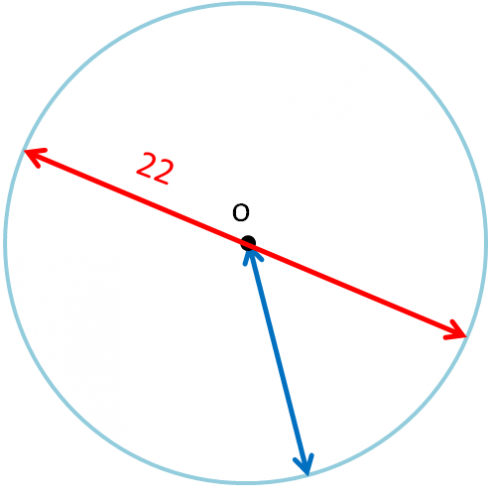# Circle Parts

In this worksheet, students must know the names of parts of circles, including radius, diameter and circumference and know that the diameter is twice the radius.Key stage:  KS 2

Curriculum topic:   Maths and Numerical Reasoning

Curriculum subtopic:   2D Shapes: Circles, Angles and Bearings

Difficulty level:### QUESTION 1 of 10

A circle can be drawn using a pair of compasses.

The distance set between the point and the pencil tip is called the radius.

The point marks the centre of the circle.The distance straight across from one edge to the other, going through the centre, is called the diameter.The diameter is twice as long as the radius.

The radius is half the length of the diameter.

When you draw a circle, the pencil line around the perimeter is called the circumference.

It is a curved line.Look at this circle.

Which letter shows the centre?a

b

c

d

Look at this circle.

Which letter shows the circumference?a

b

c

d

Look at this circle.a

b

c

d

Look at this circle.

Which letter shows the diameter?a

b

c

d

Look at this circle.

What is the name of the point labelled o ?Look at this circle.

What is the name of the line labelled r ?Look at this circle.

What is the name of the line labelled d ?Look at this circle.

What is the name of the line labelled c ?Look at this circle.

The radius is 5 units long.

How long is the diameter?

(Just write the number.)Look at this circle.

The diameter is 22 units long.

(Just write the number.)• Question 1

Look at this circle.

Which letter shows the centre?a
EDDIE SAYS
The centre is in the middle.
• Question 2

Look at this circle.

Which letter shows the circumference?d
EDDIE SAYS
The circumference is the perimeter.
• Question 3

Look at this circle.b
EDDIE SAYS
The radius goes from the centre to the circumference.
• Question 4

Look at this circle.

Which letter shows the diameter?c
EDDIE SAYS
The diameter goes from edge to edge through the centre.
• Question 5

Look at this circle.

What is the name of the point labelled o ?centre
the centre
EDDIE SAYS
The centre is the point in the middle.
• Question 6

Look at this circle.

What is the name of the line labelled r ?EDDIE SAYS
The radius goes from the centre to the circumference.
• Question 7

Look at this circle.

What is the name of the line labelled d ?diameter
the diameter
EDDIE SAYS
The diameter goes from edge to edge through the centre.
• Question 8

Look at this circle.

What is the name of the line labelled c ?circumference
the circumference
EDDIE SAYS
The circumference is the line around the edge.
• Question 9

Look at this circle.

The radius is 5 units long.

How long is the diameter?

(Just write the number.)10
EDDIE SAYS
The diameter is double the length of the radius.
• Question 10

Look at this circle.

The diameter is 22 units long.

(Just write the number.)11
EDDIE SAYS
The radius is half the length of the diameter.
---- OR ----

Sign up for a £1 trial so you can track and measure your child's progress on this activity.

### What is EdPlace?

We're your National Curriculum aligned online education content provider helping each child succeed in English, maths and science from year 1 to GCSE. With an EdPlace account you’ll be able to track and measure progress, helping each child achieve their best. We build confidence and attainment by personalising each child’s learning at a level that suits them.

Get started